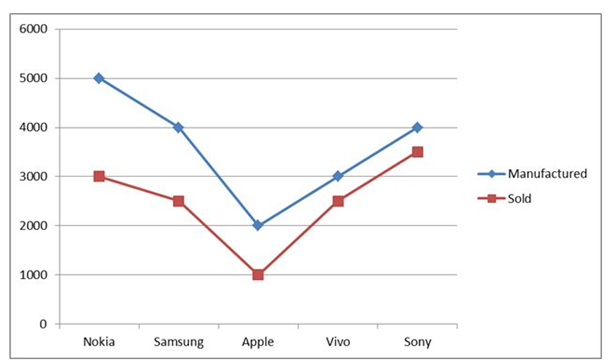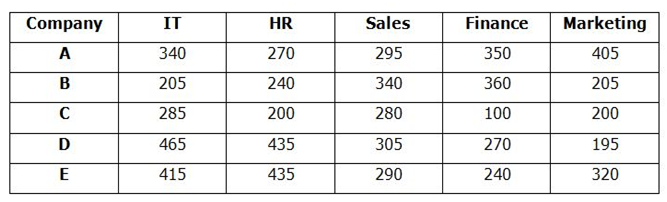# RRB PO Prelims Quantitative Aptitude (Day-05)

Dear Aspirants, Our IBPS Guide team is providing new series of Quantitative Aptitude Questions for RRB PO 2020 Prelims so the aspirants can practice it on a daily basis. These questions are framed by our skilled experts after understanding your needs thoroughly. Aspirants can practice these new series questions daily to familiarize with the exact exam pattern and make your preparation effective.

Start Quiz

Data Interpretation

Direction (1-5): Study the following information carefully and answer the questions given below.

The given line graph shows the number of mobiles manufactured and sold in five different companies in 2018.1) The number of Nokia and Sony mobile sold together is approximately what percent of the total number of mobiles manufactured in Nokia and Apple together?

A) 87%

B) 90%

C) 93%

D) 96%

E) 101%

2) What is the difference between the average number of mobile manufactured in Samsung, Apple and Vivo together and the average number of mobile sold in Vivo, Samsung and apple together?

A) 800

B) 1000

C) 1500

D) 2000

E) None of these

3) What is the average number of mobile unsold in all the companies together?

A) 1000

B) 1100

C) 1200

D) 1300

E) None of these

4) The number of unsold Sony mobile is approximately what percent of the total number of manufactured in Samsung mobile?

A) 12.5%

B) 18.5%

C) 22.5%

D) 25%

E) None of these

5) What is the ratio of the number of mobile sold in Apple and Nokia together to the number of mobile sold in Sony?

A) 6:5

B) 7:6

C) 8:7

D) 9:8

E) None of these

Data Interpretation

Direction (6-10): Study the following information carefully and answer the questions given below.

The given table shows the number of employees in five different companies in five different departments – IT, HR, Sales, Finance and Marketing.6) What is the difference between the average number of IT department employees from E and C together and the average number of Finance department employees from A and B together?

A) 10

B) 8

C) 7

D) 5

E) None of these

7) What is the ratio of the total number employees from D in all the departments together to the total number of employees from B in all the departments together?

A) 167:135

B) 165:133

C) 168:139

D) 14:11

E) None of these

8) What is the average number of Marketing department employees in all the companies together?

A) 245

B) 255

C) 265

D) 275

E) None of these

9) The number of HR department employees from E is approximately what percent of the total number of employees from A in all the departments together?

A) 26%

B) 28%

C) 30%

D) 32%

E) None of these

10) What is the difference between the total number of HR and Sales department employees from all the companies together?

A) 50

B) 60

C) 70

D) 80

E) None of these

Directions (1-5) :

Required percentage = (3000 + 3500)/(5000 + 2000) * 100

= 93%

Difference = (4000 + 2000 + 3000)/3 – (2500 + 2500 + 1000)/3

=3000 – 2000

=1000

Unsold Nokia mobile = 5000 – 3000 = 2000

Unsold Samsung mobile = 4000 – 2500 = 1500

Unsold Apple mobile = 2000 – 1000 = 1000

Unsold Vivo mobile = 3000 – 2500 = 500

Unsold Sony mobile = 4000 – 3500 = 500

Average = (2000 + 1500 + 1000 + 500 + 500)/5

= 1100

Unsold Sony mobile = 4000 – 3500 = 500

Required percentage = (500/4000) * 100 = 12.5%

Required ratio = (1000 + 3000):3500

= 8:7

Directions (6-10) :

Average of IT department employees from C and E = (285 + 415)/2 = 350

Average of Finance department employee from A and B = (350 + 360)/ 2= 355

Difference = 355 – 350 = 5

Required ratio = (465 + 435 + 305 + 270 + 195): (205 + 240 + 340 + 360 + 205)

= 1670: 1350

= 167: 135

Average = (405 + 205 + 200 + 195 + 320)/5

= 265

Required ratio = 435/(340 + 270 + 295 + 350 + 405) * 100

= 26%

HR = 270 + 240 + 200 + 435 + 435 = 1580

Sales = 295 + 340 + 280 + 305 + 290 = 1510

Difference = 1580 – 1510 = 70

 Check Here to View RRB PO Prelims 2020 Quantitative Aptitude Questions Day – 05 Day – 04 Day – 03 Click Here for SBI Clerk 2020 – Detailed Exam Notification